# 深度学习基础——感知机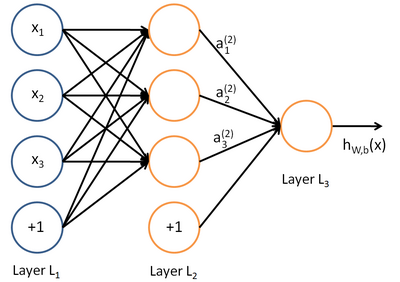# 感知器——神经网络的组成单元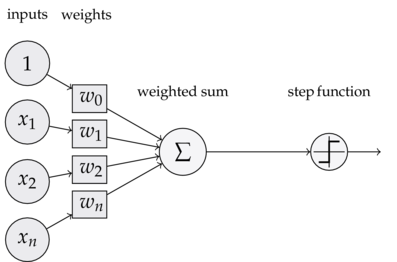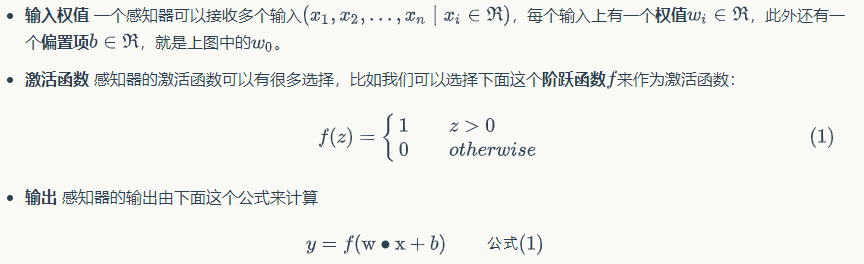### 举例：用感知器实现and函数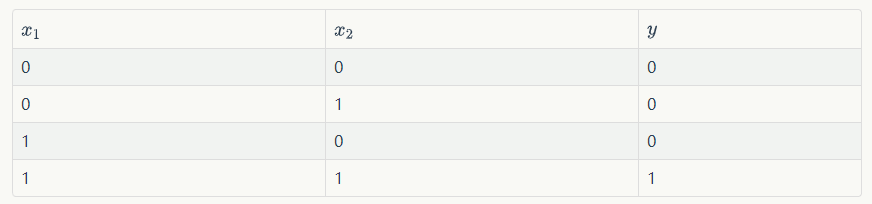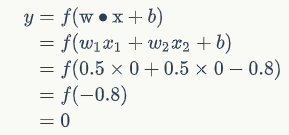### 举例2：用感知器实现or函数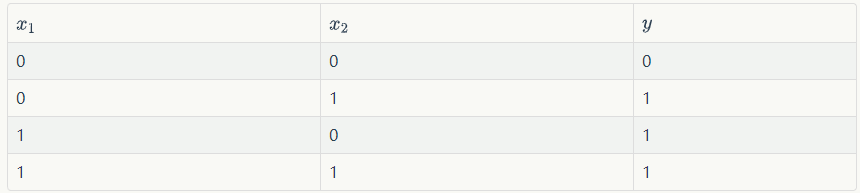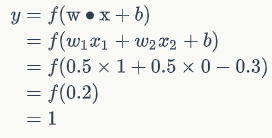# 感知器的其他功能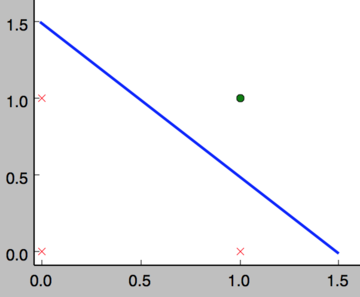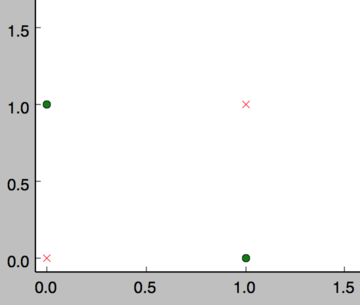# 感知器训练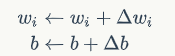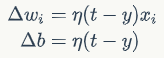wi是与输入xi对应的权重项，b是偏置项。事实上，可以把b看作是值永远为1的输入xb所对应的权重。t是训练样本的实际值，一般称之为label。而y是感知器的输出值，它是根据公式(1)计算得出。$\eta$是一个称为学习速率的常数，其作用是控制每一步调整权的幅度。

# 编程实战：实现感知器

class Perceptron(object):
def __init__(self, input_num, activator):
'''
初始化感知器，设置输入参数的个数，以及激活函数。
激活函数的类型为double -> double
'''
self.activator = activator
# 权重向量初始化为0
self.weights = [0.0 for _ in range(input_num)]
# 偏置项初始化为0
self.bias = 0.0
def __str__(self):
'''
打印学习到的权重、偏置项
'''
return 'weights\t:%s\nbias\t:%f\n' % (self.weights, self.bias)
def predict(self, input_vec):
'''
输入向量，输出感知器的计算结果
'''
# 把input_vec[x1,x2,x3...]和weights[w1,w2,w3,...]打包在一起
# 变成[(x1,w1),(x2,w2),(x3,w3),...]
# 然后利用map函数计算[x1*w1, x2*w2, x3*w3]
# 最后利用reduce求和
return self.activator(
reduce(lambda a, b: a + b,
map(lambda (x, w): x * w,
zip(input_vec, self.weights))
, 0.0) + self.bias)
def train(self, input_vecs, labels, iteration, rate):
'''
输入训练数据：一组向量、与每个向量对应的label；以及训练轮数、学习率
'''
for i in range(iteration):
self._one_iteration(input_vecs, labels, rate)
def _one_iteration(self, input_vecs, labels, rate):
'''
一次迭代，把所有的训练数据过一遍
'''
# 把输入和输出打包在一起，成为样本的列表[(input_vec, label), ...]
# 而每个训练样本是(input_vec, label)
samples = zip(input_vecs, labels)
# 对每个样本，按照感知器规则更新权重
for (input_vec, label) in samples:
# 计算感知器在当前权重下的输出
output = self.predict(input_vec)
# 更新权重
self._update_weights(input_vec, output, label, rate)
def _update_weights(self, input_vec, output, label, rate):
'''
按照感知器规则更新权重
'''
# 把input_vec[x1,x2,x3,...]和weights[w1,w2,w3,...]打包在一起
# 变成[(x1,w1),(x2,w2),(x3,w3),...]
# 然后利用感知器规则更新权重
delta = label - output
self.weights = map(
lambda (x, w): w + rate * delta * x,
zip(input_vec, self.weights))
# 更新bias
self.bias += rate * delta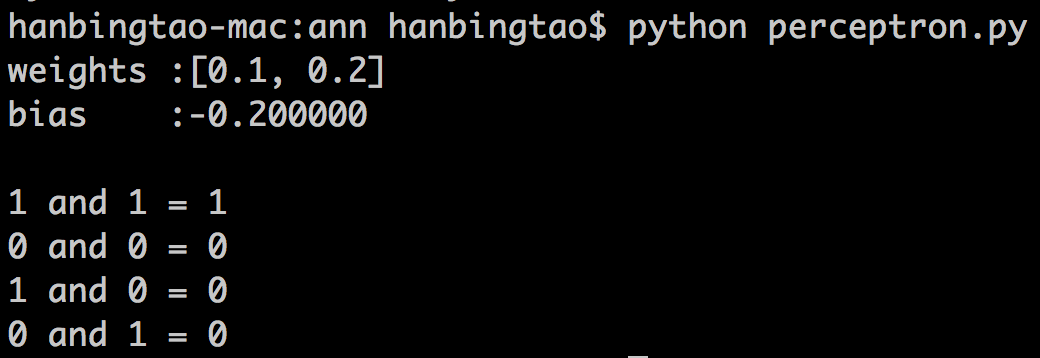# 多层感知机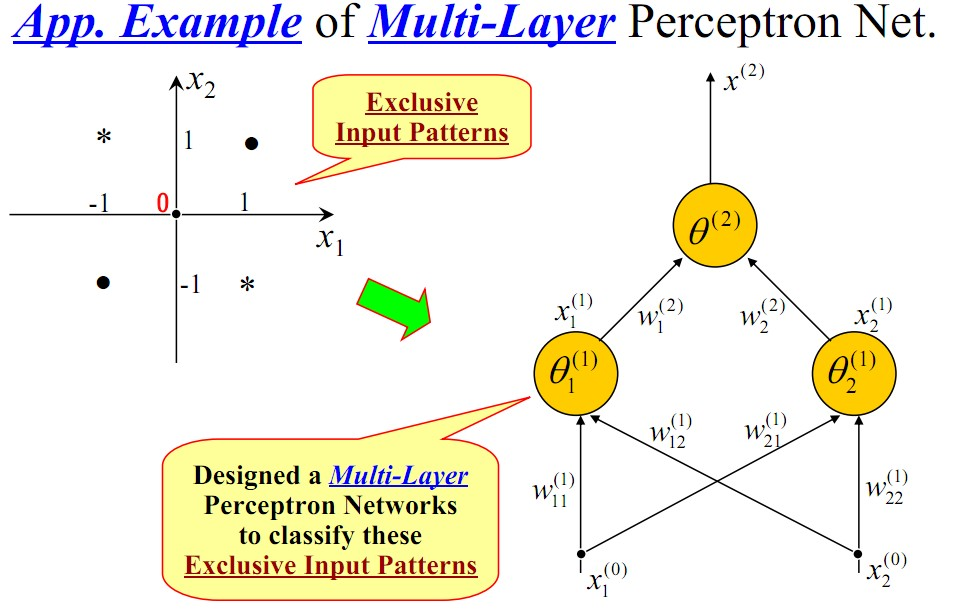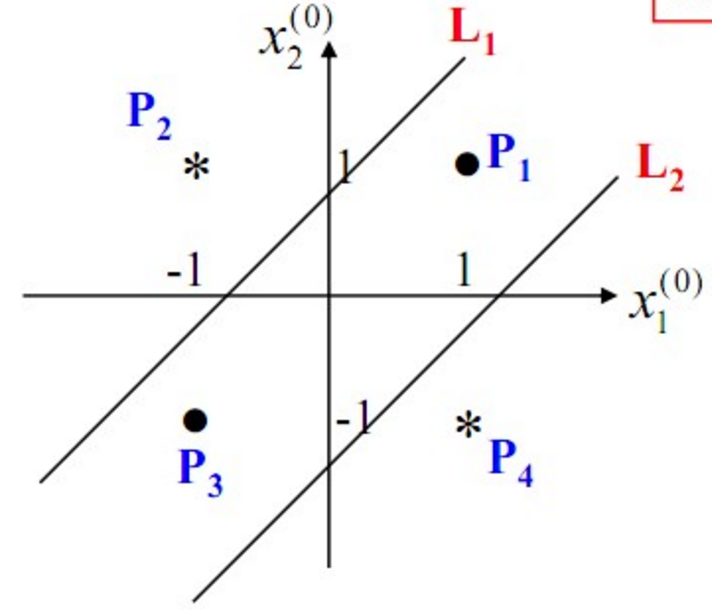posted @ 2019-08-01 15:31  Shaw_喆宇  阅读(11181)  评论(0编辑  收藏